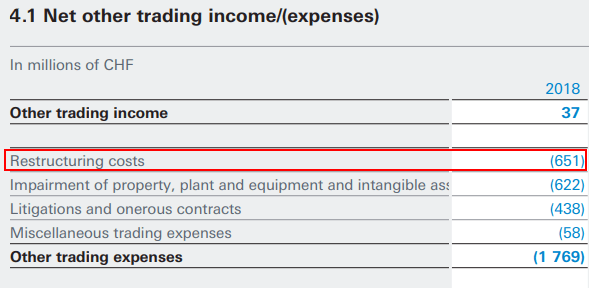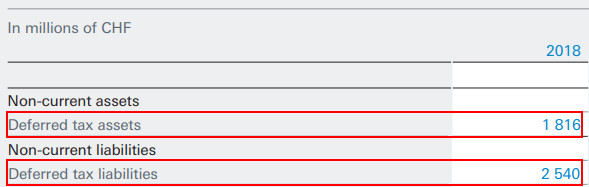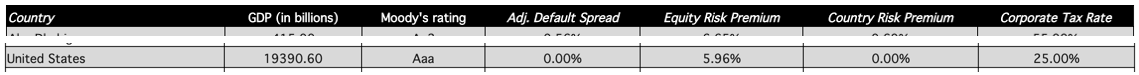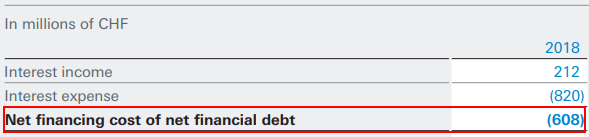## Introduction

In today’s post, we are doing a short case study, trying to calculate the Economic Value Added (EVA) for Nestlé for FY 2018, using actual data available in the public domain.

Nestlé is the world’s biggest food and beverage company, with more than 2000 brands, present in 190 countries worldwide, generating more than 90 billion dollars in revenue in 2018.

We will see how we can apply the theory we looked at in the EVA article in a real-life scenario.

There’s a summary of all information sources within the case study at the end.

Let’s look at the EVA formula and start calculating the components:## Calculating Nestlé’s NOPAT

To calculate Nestlé ‘s Net Operating Profit After Tax (NOPAT), we will look into their Income Statement for FY 2018. Financial Statements of Nestlé can be found here:

Nestlé Consolidated Financial Statements for FY 2018

Here’s an excerpt of the information we need to calculate NOPAT:Operating Profit of Nestlé for 2018 is 13,752 mil CHF.

This profit contains non-cash items like Restructuring Costs, Depreciation, and Amortization, etc. We assume depreciation and amortization are the same for accounting and reporting purposes, so no adjustment to NOPAT is required.

However, we need to adjust for Restructuring Costs.

We can find these from Nestlé’s Financial statements:Nestlé’s Restructuring Costs for 2018 are 651 mil CHF. We can calculate the Adjusted Operating Profit:

`Adjusted Operating Profit = 13,752 + 651 = 14,403 mil CHF`

To calculate NOPAT, we also need the effective tax rate, which we can calculate from the Income Statement in the Financial Statements:

`Effective tax rate = Taxes / Profit before taxesEffective tax rate = 3,439 / 12,991 = 26.40%`

Now we can calculate NOPAT itself:

`NOPAT = Adjusted Operating Profit * (1 – Effective Tax Rate)NOPAT = 14,403 * (1 – 26.40%)NOPAT = 14,403 * 0.736 = 10,600.608 ~ 10,601 mil CHF`

## Calculating Nestlé’s Invested Capital

Invested capital is the actual value of debt and equity invested in the company.

### Total Debt

Here’s an excerpt from the Consolidated Statement of Financial Position (Balance Sheet) of Nestlé for FY 2018:`Total Debt = Loans Payable + Current Portion of Long-term Debt + Long-term DebtTotal Debt = 0 + 14,695 + 25,700 = 40,395 mil CHF`

### Equity

Here’s an excerpt from the Consolidated Statement of Financial Position (Balance Sheet) of Nestlé for FY 2018:`Adjusted Equity     = Shareholders equity     + Net Deferred Tax     + Non-controlling Interest     + Accumulated Other Comprehensive (income)/expenses`

We need to calculate Net deferred tax, which is the difference between Deferred tax assets and liabilities. We can find information on those in the Consolidated Statement of Financial Position (Balance Sheet):`Net deferred tax = 1,816 – 2,540 = (724) mil CHF`

Knowing that, we can go back and calculate Adjusted Equity and from there Invested Capital:

`Adjusted Equity = 57,363 + (724) + 1,040 + 0 = 57,679 mil CHFInvested Capital = Total Debt + Adjusted Equity = 40,395 + 57,679 = 98,074 mil CHF`

## Calculating Nestlé’s WACC

To calculate Nestlé’s Weighted Average Cost of Capital (WACC), we need to look into the Cost of Equity and Cost of Debt for the company.

### Cost of EquityFrom the Financial Statements of Nestlé, we can see that the issued and fully paid capital consists of 3,063,000,000 shares, 0.10 CHF each share.

Yahoo Finance Summary on NestléThe current market price as of 27th September 2019, is 108.55 USD.

`Market capitalization = Number of Shares * Market priceMarket capitalization = 3,063,000,000 * 108.55Market capitalization = 332.49 bil USD`Applying the exchange rate we get:

`Market capitalization of Equity = 329.20 bil CHF`

Let’s calculate the cost of equity with the CAPM approach:

`Cost of Equity = Risk-free rate + Beta * Risk Premium`

First, we get the ten-year treasury constant maturity, as we consider treasury securities risk-free since they are backed by the U.S. government.

Bankrate’s Ten-Year Treasury Constant MaturityThe risk-free rate is 1.64%.

The next component of the Cost of Equity calculation is the Risk Premium, which can be found in a publication by Aswath Damodaran on the Leonard N. Stern School of Business website:Nestlé is a Swiss company; however we can assume they perform most of their business in the U.S. In such a case, it might be better to work with the Risk Premium for the U.S. instead of the one for Switzerland. Incidentally, the Equity Risk Premium for both the United States and Switzerland is 5.96% at the time of performing the case study.

The next metric we need is Nestlé’s Beta coefficient. If we look back at the financial data from Yahoo Finance, we can see that it’s 0.41.

We now have all the components to calculate the Cost of Equity:

`Cost of Equity = Risk-free rate + Beta * Risk PremiumCost of Equity = 1.64% + 0.41 * 5.96%Cost of Equity = 4.08%`

### Cost of Debt

Next, we need the net finance costs, to calculate the Cost of Debt. We can find information on these in the Consolidated Financial Statements:Net Interest Cost for the year is 608 mil CHF. We already calculated the value of Total Debt at 40,395 mil CHF.

`Effective Interest Rate = Net financing costs / DebtEffective Interest Rate = 608 / 40,395 = 1.5%`

Now we have all the required metrics to calculate the Weighted Average Cost of Capital (WACC) of Nestlé.

`WACC = Equity / (Equity + Debt) * Cost of Capital       + Debt / (Equtiy + Debt) * Cost of Debt * (1 – Tax Rate)WACC = 329,200 / 369,595 * 4.08% + 40,395 / 369,595 * 1.5% * (1 – 26.4%)WACC = 0.03634 + 0.00121 = 0.03755 = 3.76%`

## EVA Calculation

Now that we have calculated all the components of the Economic Value Added, we can perform the EVA calculation itself:

`EVA = NOPAT – WACC * Invested CapitalEVA = 10,601 – 3.76% * 98,074 = 6,913 mil CHF`

Nestlé has a positive EVA for 2018 at the amount of 6,913 mil CHF. The amount represents the value of wealth that the company has generated for its shareholders this year, making it a worthy investment.

## Information Sources

1. Consolidated Financial Statements of Nestle for FY 2018 – https://www.nestle.com/sites/default/files/asset-library/documents/library/documents/financial_statements/2018-financial-statements-en.pdf
2. Yahoo Finance Summary for Nestle – https://finance.yahoo.com/quote/NSRGY/?guccounter=1&guce_referrer=aHR0cHM6Ly93d3cuZ29vZ2xlLmNvbS8&guce_referrer_sig=AQAAAB6rWiPs-L8nGhcSwBJptDAp3btRHZ8VrsdXC7tomb01uyPteeAYYr9Atr1kxE7amAuA7NzaYVXEc6PwY56oO9E5URy0YN7ROgxvcaZA2fmwJzIAVtS4zHtpr7YEsROPTS-xTjSoWhRTUTXXn8xf8S2PYX1gLH4txcimH0iBojPS
3. Bankrate’s ten-year treasury note rate – https://www.bankrate.com/rates/interest-rates/10-year-treasury-bill.aspx## FCCA, FMVA

Hi! I am a finance professional with 10+ years of experience in audit, controlling, reporting, financial analysis and modeling. I am excited to delve deep into specifics of various industries, where I can identify the best solutions for clients I work with.

In my spare time, I am into skiing, hiking and running. I am also active on Instagram and YouTube, where I try different ways to express my creative side.